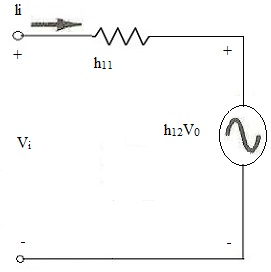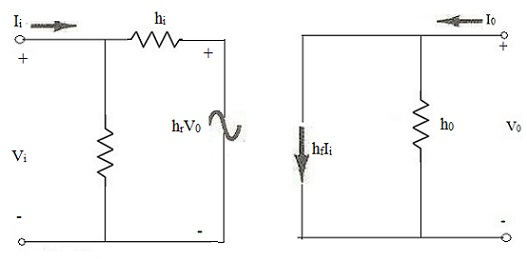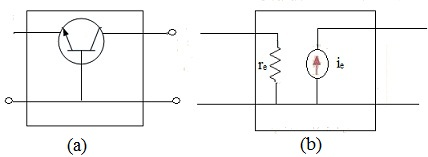#### Hybrid Equivalent Model, Physics tutorial

h-parameters:

These are four constants that explained behavior of two-port linear network. Linear network is one in which resistance; inductance and capacitance remain fixed when voltage across them is changed.

Electrical behavior of such a circuit can be explained with the help of four hybrid parameters or constants designated as h11, h12, h21, h22. In this kind of double-number subscript, it is implied that first variable is always divided by other. Quantity 1 refers to quantity on input side and 2 to quantity on output side. Letter 'h' has come from word hybrid that signifies mixture of noticeably different items. These constants are hybrid as they have different units.

Hybrid Equivalents Model:

The quantities hie, hre, hfe, and hoe are known as hybrid parameters from the word hybrid and are components of small-signal equivalent circuit to be described shortly. Description of hybrid equivalent model will start with general two-port system. The following set of equations is only one of a number of ways in which four variables can be related. It is the most frequently used in transistor circuit analysis, though, and thus is discussed in details.

Vi = h11Ii + h12Ve

I0 = h21Ii + h22V0

h11 = Vi/Ii|V0 = 0 ohms

Ratio indicates that parameter h11 is impedance parameter with units of ohms. As it is ratio of input voltage to input current with output terminals shorted, it is known as short- circuit input-impedance parameter. Subscript 11 of h11 defines fact that parameter is computed by ratio of quantities measured at input terminals.

If Ii is set equal to zero by opening input leads, will result for h12 is given below:

h12 = Vi/Vo|I0 = 0 unit

Parameter h12, thus, is ratio of input voltage to output voltage with input current set to zero. It has no units as it is ratio of voltage levels and is known as open-circuit reverse transfer voltage ratio parameter.

If, Vo is equal to zero by again shorting output terminals, result for h21 is given below:

h21 = I0/Ii|V0 = 0 unit less

Parameter h21 is ratio of output current to input current with output terminals shorted. It is known as short circuit forward transfer current ratio parameter: and it is unit less.

Last parameter, h22, can be estimated by again opening input leads to set II = 0 and solving for h22:

h22 = I0/V0|Ii = 0 Siemens

As it is ratio of output current to output voltage, it is output conductance parameter and is estimated in Siemens (S). It is thus known as open-circuit output admittance parameter.

Hybrid Input Equivalent Circuit:As each term of equation has unit of current, now apply Kirchhoff's current law in reverse to get circuit. As h22 has unit of admittance, which for transistor model is conductance, it is signified by resistor symbol. Though, that resistance in ohms of this resistor is equal to reciprocal of conductance(1/h22)

Complete Hybrid Equivalent Circuit:

Complete ac equivalent circuit for basic three-terminal linear device is indicated with new set of subscripts for h- parameters.The notation is of more practical nature as it relates h-parameters to resulting ratio. Using h-parameter for three terminal devices (transistor circuits), their numerical subscript are replaced by first letters for defining them:

h11 =hi = input impedance

h12 = hf = forward current gain

h21 = hr = reverse voltage gain

h22 = ho = output impedance

The second subscript is added to above parameters to point out particular configuration. For instance, for common-emitter connection, four parameters are written as: hie hfe hre and hoe likewise, for common-base and common-collector connections, these are written as hib, hfb, hrb, hob, and hic, hfc, hrc, hoc respectively.

When small signals are involved, transistor behaves like linear device as its output ac signals differs directly as input signal. Therefore for small ac signals, every transistor has its own characteristics set of h-parameters or constants.

The h-parameter depends on a number of factors such as:

• Transistor type
• Configuration
• Operating point
• Temperature
• Frequency

These parameters can be found experimentally or graphically. The parameters hi and hr are determined from the input characteristics of the common-emitter transistors wheras hf and ho are found from the output characteristics.Figure (a) given above shows a common-base transistor connected in a black box. Figure (b) gives its equivalent circuit. It must be noted that no external biasing resistor or any signal source is joined to transistor. Two forward h-parameters can be found from circuit where short has been put across output. Input impedance is simply re.

Therefore hib = re

Output current equals input current i.e. as it flows out of box, it is taken as negative. Forward current gain is:

hfb = -ie/ie = α ≈ -1

Also, reverse parameters can be found from circuit diagram. When input terminals are open, there can be no ac emitter current. It means that ac current source (inside box) has value of zero and so appears as open. Due to this open, no voltage can appear across input terminals, though, large V2 may be. Hence V1 = 0.

Tutorsglobe: A way to secure high grade in your curriculum (Online Tutoring)

Expand your confidence, grow study skills and improve your grades.

Since 2009, Tutorsglobe has proactively helped millions of students to get better grades in school, college or university and score well in competitive tests with live, one-on-one online tutoring.

Using an advanced developed tutoring system providing little or no wait time, the students are connected on-demand with a tutor at www.tutorsglobe.com. Students work one-on-one, in real-time with a tutor, communicating and studying using a virtual whiteboard technology.  Scientific and mathematical notation, symbols, geometric figures, graphing and freehand drawing can be rendered quickly and easily in the advanced whiteboard.

Free to know our price and packages for online physics tutoring. Chat with us or submit request at info@tutorsglobe.com